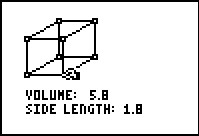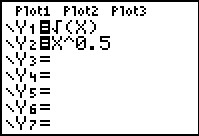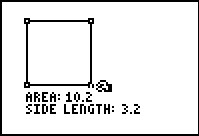Algebra II: Living on the Edge
by Texas Instruments#### Overview

Students find the edge length of an octahedron when given its volume.

#### Key Steps

•Students build a solution to a rather complex problem—finding the edge length of an octahedron given its volume—by solving two simpler problems.

First, students find a formula for the edge length of a square given its area.

•After testing this formula by comparing points on its graph with the measurements of a model square, they write an equivalent formula with fractional exponents and substitute values to find the edge length of a particular square. Students will repeat this process for the formula for the edge length of a cube given its volume.

•Finally, students solve an equation involving several different radical expressions to find a formula for the edge length of an octahedron given its volume, and use it to solve the original problem.